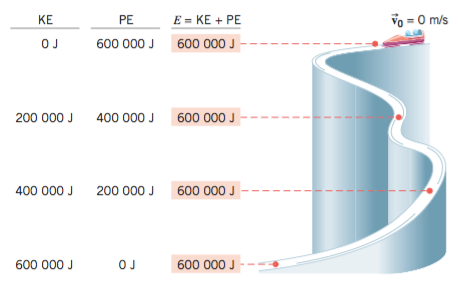top of page
Search
• Yuzhe

# The Principle of Conservation of Mechanical Energy Mechanical Energy - physics/物理

The total mechanical energy (E = KE + PE) of an object remains constant as the object moves, provided that the net work done by external nonconservative forces is zero, Wnc 0 J.Figure above shows such transformations of energy for a bobsled run, assuming that nonconservative forces, such as friction and wind resistance, can be ignored. The normal force, being directed perpendicular to the path, does no work. Only the force of gravity does work, so the total mechanical energy E remains constant at all points along the run.

#Physics

bottom of page